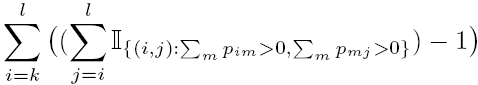# Jenkins et al. (2006)

Here you can find additional files associated with the paper "How many transcripts does it take to reconstruct the splice graph?" (Jenkins et al. 2006). Each file is described below. They are available together in this zip file.

• `ratios.xls` contains likelihood ratio tests for the pairwise versus in-out models applied to all 89 genes with more than 5000 putative transcripts, formatted as follows:

 Region 1 ... Total all regions Ensembl ID Start of region (fragment number) End of region (fragment number) Gamma Degrees of freedom p-value (%)

• `readwg.m` reads a directed ASG into Matlab. The ASG file should be formatted as in the example `ABCB5.wg` and in the same directory.
• `ABCB5.wg` is an example weighted ASG file. ‘#’ acts as a comment character. Size of graph should be number of exon fragments + 2 (representing the appended source and sink). Below each node’s outdegree is a list of destination nodes and the supporting number of ESTs. There should also be a Y/N string indicating whether this node is attached to the next one (i.e. this and the following node are part of the same exon).
• `pairwise.m` simulates in Matlab the sampling of transcripts under the pairwise model as described in the paper. Execute this after reading in an ASG using `readwg.m`. It creates an `n` x #exon-fragments matrix called `transcripts` (`n` is specified on input). Each row provides a random sample from the gene under the pairwise model. `transcripts(i,j)` is an indicator for whether exon fragment j is contained in sample i.
• `inout.m` simulates in Matlab the sampling of transcripts under the in-out model as described in the paper. It operates in the same way as `pairwise.m`.
• `maxmin.m` creates variables `maximum_transcripts` and `minimum_transcripts` which are the maximum and minimum number of informative transcripts obtainable from the current ASG. These values are calculated as described in the path-covering algorithms described in the paper. The current ASG is read into Matlab using `readwg.m`.
• `ratio.m` performs the likelihood ratio test described in the paper on the current ASG, comparing the pairwise versus in-out models. It creates variables `regionstart`, `regionend`, `Gam`, `z` and `pvalue` which are the rows of the table template above. The current ASG is read into Matlab using `readwg.m`. Determining the degrees of freedom under the pairwise model is not entirely straightforward. For a test on exons k,...,l we used the following as a sensible choice for the degrees of freedom under the pairwise model:i.e. to describe all possible outgoing destinations from a given exon i we require the number of parameters to be equal to the number of downstream acceptor sites, minus 1. The degrees of freedom is the sum of all such i over k,...,l.
• `putative_transcripts.m` returns the number of putative transcripts (i.e. distinct paths through the ASG) associated with the current ASG, which has been read into Matlab using `readwg.m`.
• `count_downstream_paths.m` is a function for use by `putative_transcripts.m`. Leave it in the same directory as this file.
• `full_asg.py` is a Python script for which returns a p-value for an ASG, based on alpha = 1. Usage:
`python full_asg.py m example.wg repeats`
where `m` is the number of transcripts we’ll draw for each repeat, and `repeat` is the number of such repeats on which the p-value is based. This operates on the ASG contained in `example.wg`. It should be executed in the same directory as `wwdigraph.py` and `digraph.py`, as well as your `example.wg`.